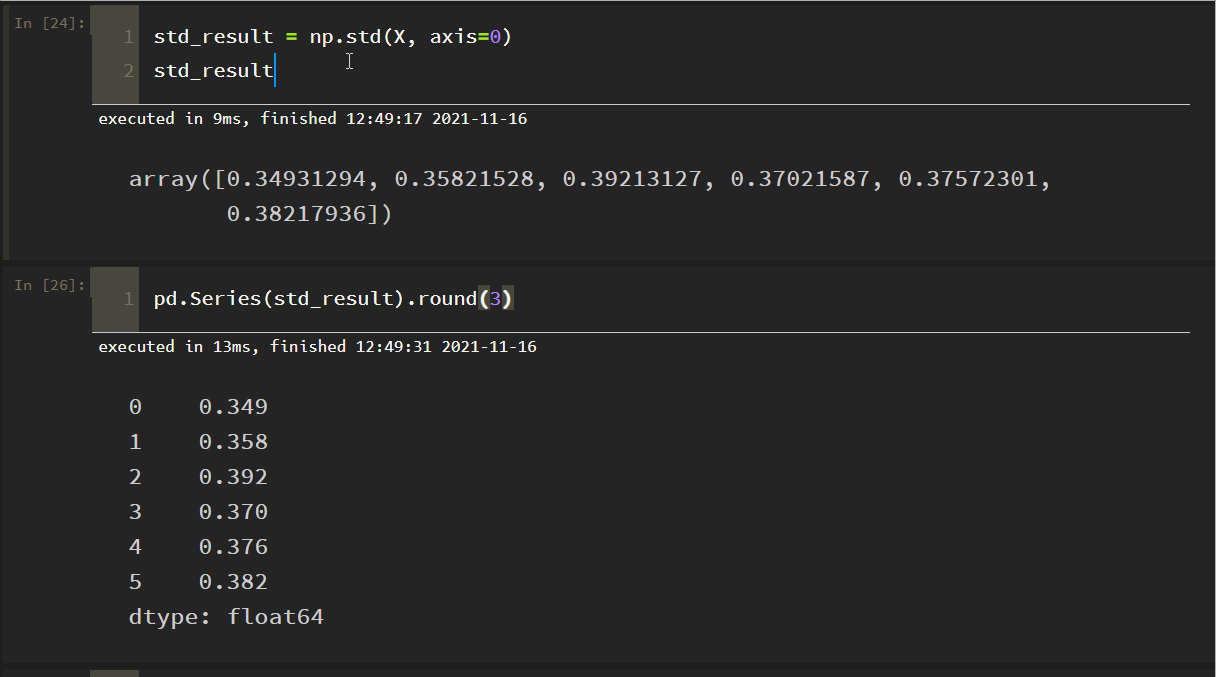﻿ Python Numpy实现计算矩阵的均值和标准差详解_python_脚本之家
python# Python Numpy实现计算矩阵的均值和标准差详解

NumPy（Numerical Python）是Python的一种开源的数值计算扩展。这种工具可用来存储和处理大型矩阵，比Python自身的嵌套列表结构要高效的多。本文主要介绍用NumPy实现计算矩阵的均值和标准差，感兴趣的小伙伴可以了解一下

## 一、前言

CRITIC权重法是一种比熵权法和标准离差法更好的客观赋权法：

• 它是基于评价指标的对比强度和指标之间的冲突性来综合衡量指标的客观权重。考虑指标变异性大小的同时兼顾指标之间的相关性，并非数字越大就说明越重要，完全利用数据自身的客观属性进行科学评价。
• 对比强度是指同一个指标各个评价方案之间取值差距的大小，以标准差的形式来表现。标准差越大，说明波动越大，即各方案之间的取值差距越大，权重会越高；Sj表示第 j 个指标的标准差，在 CRITIC 权重法中使用标准差来表示各指标的内取值的差异波动情况，标准差越大表示该指标的数值差异越大，越能放映出更多的信息，该指标本身的评价强度也就越强，应该给该指标分配更多的权重。## 二、详解计算均值和标准差

```a = np.array([
[1, 2, 3],
[4, 5, 6],
[7, 8, 9]
])
a
```

```print("整体的均值：", np.mean(a))              # 整体的均值
print("每一列的均值：", np.mean(a, axis=0))    # 每一列的均值
print("每一行的均值：", np.mean(a, axis=1))    # 每一行的均值
```

```print("整体的方差：", np.std(a))              # 整体的标准差
print("每一列的方差：", np.std(a, axis=0))    # 每一列的标准差
print("每一列的方差：", np.std(a, axis=1))    # 每一行的标准差
```## 三、实践：CRITIC权重法计算变异系数

```import numpy as np
import pandas as pd
```

```df = pd.read_excel("./datas/result03.xlsx")
df

datas = df.iloc[:, 1:]
datas
``````X = datas.values
xmin = X.min(axis=0)
xmax = X.max(axis=0)
xmaxmin = xmax - xmin
n, m = X.shape
print(m, n)
for i in range(n):
for j in range(m):
if j == 5:
X[i, j] = (xmax[j] - X[i, j]) / xmaxmin[j]   # 越小越好
else:
X[i, j] = (X[i, j] - xmin[j]) / xmaxmin[j]   # 越大越好

X = np.round(X, 5)
print(X)
```